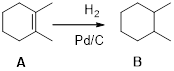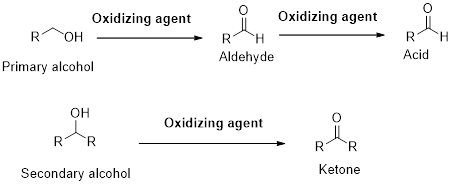Chapter 23, Problem 97GQ

Chapter
Section
Textbook Problem

Identify the reaction products, and write an equation for the following reactions of CH2=CHCH2OH.(a) H2 (hydrogenation, in the presence of a catalyst)(b) Oxidation (excess oxidizing agent)(c) Addition polymerization(d) Ester formation, using acetic acid

(a)

Interpretation Introduction

Interpretation:

The products and chemical equation has to be written for the reactions of CH2=CHCH2OH with H2(hydrogenation, in presence of a catalyst).

Concept Introduction:

Hydrogenation:

Palladium on carbon (Pd/C) is used as a catalyst for hydrogenation of alkenes and alkanes.In general, an alkene undergo hydrogentaion reaction when it is treated with hydrogen gas. The addition of two hydrogen atoms takes place on the carbon-carbon double bond.

Explanation

The product obtained after hydrogenation reaction of given compound is a primary alcohol. The equation for the hydrogenation reaction is written below.

(b)

Interpretation Introduction

Interpretation:

The products and chemical equation has to be written for the reactions of CH2=CHCH2OH with excess oxidizing agent.

Concept Introduction:

A primary alcohol gives carboxylic acid (RCOOH) in presence of excess oxidizing agent. However, a secondary alcohol gives ketone in presence of excess oxidizing agent. A tertiary alcohol does not undergo oxidation reaction.(c)

Interpretation Introduction

Interpretation:

The products and chemical equation has to be written for the reactions of CH2=CHCH2OH with Addition polymerization.

Concept Introduction:

The polymerisation reaction of given monomer CH2=CHCH2OH unit gives polymeized product. The product is an addition polymer as during polymerisation its monomer units combine directly.

(d)

Interpretation Introduction

Interpretation:

The products and chemical equation has to be written for the reactions of CH2=CHCH2OH with acetic acid forms an ester.

Concept Introduction:

Esters are prepared by the condensation reaction of carboxylic acids and alcohols. One mole of each acid and alcohol undergo condensation reaction which produce one mole of ester.

Still sussing out bartleby?

Check out a sample textbook solution.

See a sample solution

The Solution to Your Study Problems

Bartleby provides explanations to thousands of textbook problems written by our experts, many with advanced degrees!

Get Started